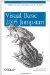# 2.7. Operators

VB 2005 supports the various operators shown in Table 2-2.

##### Table 2-2. Operators supported in VB 2005

Type

Language element

Description

Arithmetic

^

Raises to the power of

Subtraction

*

Multiplication

/

Division

\

Integer Division

Mod

Modulus (remainder)

+

Assignment

=

Assigns a value to a variable or property

^=

Raises the value of a variable to the power of an expression and assigns the result back to the variable (new in VB 2005)

*=

Multiplies the value of a variable by the value of an expression and assigns the result to the variable (new in VB 2005)

/=

Divides the value of a variable by the value of an expression and assigns the result to the variable (new in VB 2005)

\=

Divides the value of a variable by the value of an expression and assigns the integer result to the variable (new in VB 2005)

+=

Adds the value of an expression to the value of a variable and assigns the result to the variable (works for strings as well) (new in VB 2005)

-=

Subtracts the value of an expression from the value of a variable and assigns the result to the variable (new in VB 2005)

&=

Concatenates a String expression to a String variable and assigns the result to the variable (new in VB 2005)

Comparison

=

Equal

<>

Not equal to

<

Less than

>

Greater than

<=

Less than or equal to

>=

Greater than or equal to

Like

Compares a string against a pattern

Is

Compares two object reference variables

IsNot

Compares two object reference variables

Concatenation

&

Concatenates two strings

+

Concatenates two strings

Logical/bitwise operations

Not

Logical negation on a Boolean expression

And

Logical conjunction on two Boolean expressions, or bitwise conjunction on two numeric expressions

Or

Logical disjunction on two Boolean expressions, or bitwise disjunction on two numeric values

Xor

Logical exclusion operation on two Boolean expressions, or bitwise exclusion on two numeric expressions

AndAlso

Short-circuiting logical conjunction on two expressions (new in VB 2005)

OrElse

Short-circuiting logical disjunction on two expressions (new in VB 2005)

Miscellaneous operations

Creates a procedure delegate instance that references the specific procedure (new in VB 2005)

GetType

Returns a Type object for the specified type (new in VB 2005)

When testing for the equality of numeric values, use the = operator. Use the Is operator to test the equality of objects. Chapter 3 will discuss the use of the Is operator in greater detail.VB 6 Tip: Of particular interest to VB 6 users is the new support for assignment operators in Visual Basic 2005. In VB 6, to increment a variable, you must write code that looks something like this: ` var = var + 1 ` In Visual Basic 2005, you can now rewrite the line as: ` var += 1 `

The IsNot operator is new in VB 2005. Often you need to negate the comparison of an object, such as:

` Dim obj As Button If Not obj Is Nothing Then    ' obj contains an object reference        .…     End If `

In this case, your code will be more readable if you use the IsNot operator:

` If obj IsNot Nothing Then        ' obj contains an object reference        .…     End If `Visual Basic 2005 Jumpstart
ISBN: 059610071X
EAN: 2147483647
Year: 2005
Pages: 86
Authors: Wei-Meng Lee

Similar book on Amazon